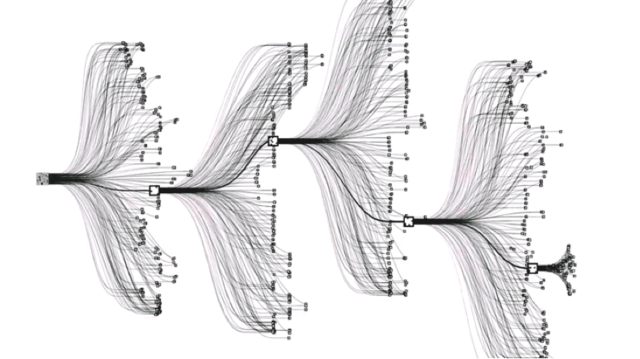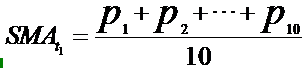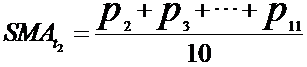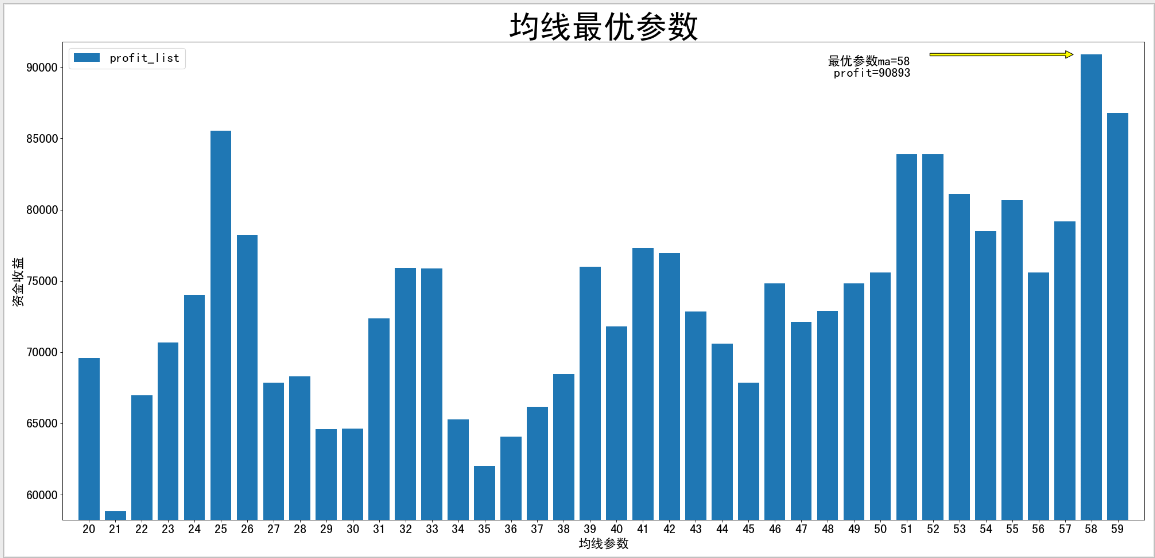# 你以为 AlphaGo 只是下围棋厉害？不，它还能用来优化金融交易策略参数## 提取阿尔法狗中的灵感

AlphaGo 则采用了深度神经网络、监督 / 强化学习、蒙特卡洛树搜索这三个“利器”的结合完美解决了以上这些问题。下面简单了解下这三个“利器”，看下是哪件“利器”解决了搜索棋局样本空间巨大这个问题。## 深入了解蒙特卡洛思想

### 实验步骤：

（1）设函数 y=f(x)=200*sin(x)e-0.05x
（2）随即取点（X），使得 -2 <=X<=2，即点在区间 [-2,2] 内
（3）通过公式 f(x)> f(x)max 判断当前点的值是否是最大值
（4）每次更新最大值和对应的点。
（5）运行结果发现极大值 185.1204262706596, 极大值点为 1.5144491499169481

def cal_extremum_mc(n = 1000000):     y_max = 0.0    x_min, x_max = -2.0, 2.0    y = lambda x:200*np.sin(x)*np.exp(-0.05*x)#匿名函数     for i in range(0, n+1):        x0 = random.uniform(x_min, x_max)        if y(x0) > y_max:            y_max = y(x0)            x_max = x0    return y_max, x_max

## 金融交易策略的参数优化def cal_moveavg_mc(n = 500):     avg1_min, avg1_max = 10, 50    avg2_min, avg2_max = 50, 90    ma_list = []     for i in range(0, n+1):        avg1 = int(random.uniform(avg1_min, avg1_max))        avg2 = int(random.uniform(avg2_min, avg2_max))        ma_list.append([avg1,avg2])    #此处添加回测采样参数    return ma_list